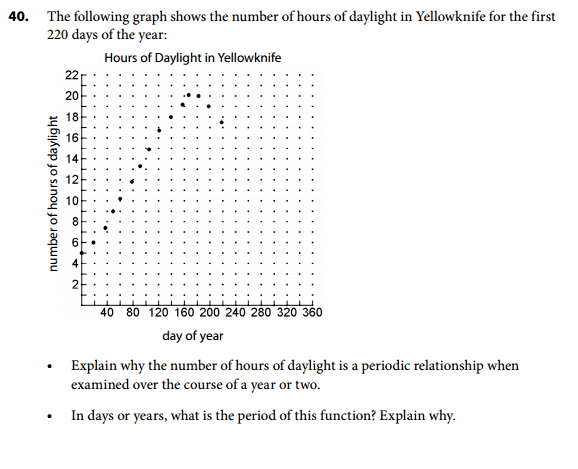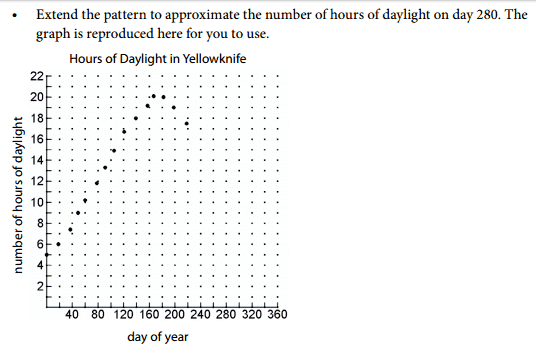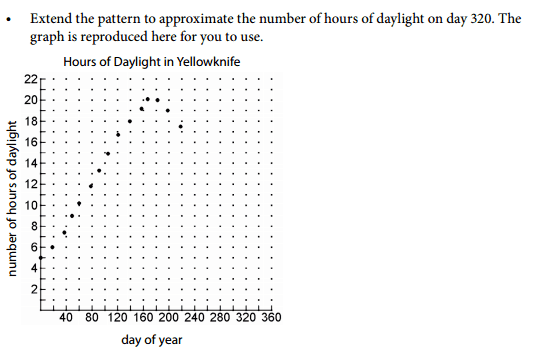# Periodic Functions Homework: Daylight Hours

• alexandria
The homework statement has three parts: a) the number of hours of daylight is a periodic relationship, because it repeats the same wave-like pattern over the course of 1-2 years; b) the period is the amount of time it takes for one cycle to occur; and c) they want me to extend the pattern to approximate the number of hours of daylight for specific days, how do i do that? do i just guess the points and then extend the graph?In summary, the period of the function is around 170 days, and the homework statement asks for someone to approximate the number of hours of daylight for specific days.

## Homework Statement## Homework Equations

no equations required

## The Attempt at a Solution

[/B]
a)

The number of hours of daylight is a periodic relationship, because it repeats the same wave-like pattern over the course of 1-2 years.

b)
the period is the amount of time it takes for one cycle to occur. So, in this case the period of this function would be around 170??

for part c) and d) they want me to extend the pattern to approximate the number of hours of daylight for specific days, how do i do that? do i just guess the points and then extend the graph?

thanks for the help :)

alexandria said:
for part c) and d) they want me to extend the pattern to approximate the number of hours of daylight for specific days, how do i do that? do i just guess the points and then extend the graph?
Have you considered that there is symmetry involved?

alexandria said:
The number of hours of daylight is a periodic relationship, because it repeats the same wave-like pattern over the course of 1-2 years.
If it repeats it is periodic. Yes. That is the definition of periodic. But that just begs the question: Does the pattern repeat or not?

What do you know about astronomy and the reason for the seasons?
the period is the amount of time it takes for one cycle to occur. So, in this case the period of this function would be around 170??
Is the day length at day 0 the same as the day length at day 170? If the function has a period of 170 days, what does that mean about the value of the function after 170 days have elapsed?

some basic information that is required in a sense to picture this problem mentally...

• Yellowknife is infact in Canada or somewhere around there.

• Canada is pretty close to north pole, in terms of map location. In terms of northern latitude.

So... when Canada has summer once per year. That summer, is when the sunlight is the highest amount. When Canada has winter that is once per year. and it is when Canada has the smallest amount of sunlight.

That is the basic idea. The months would be of course around december is probably the smallest amount of sunlight in Canada. In the summer months of July (sometime early July) the sunlight in Canada is at the highest levels.

Summer and winter are on opposite sides separated by 183 days between each other.
It looks like this corresponds exactly with the graph that you provided.

Ymax (sunlight) is reached when the x-axis goes to 180 days (roughly)